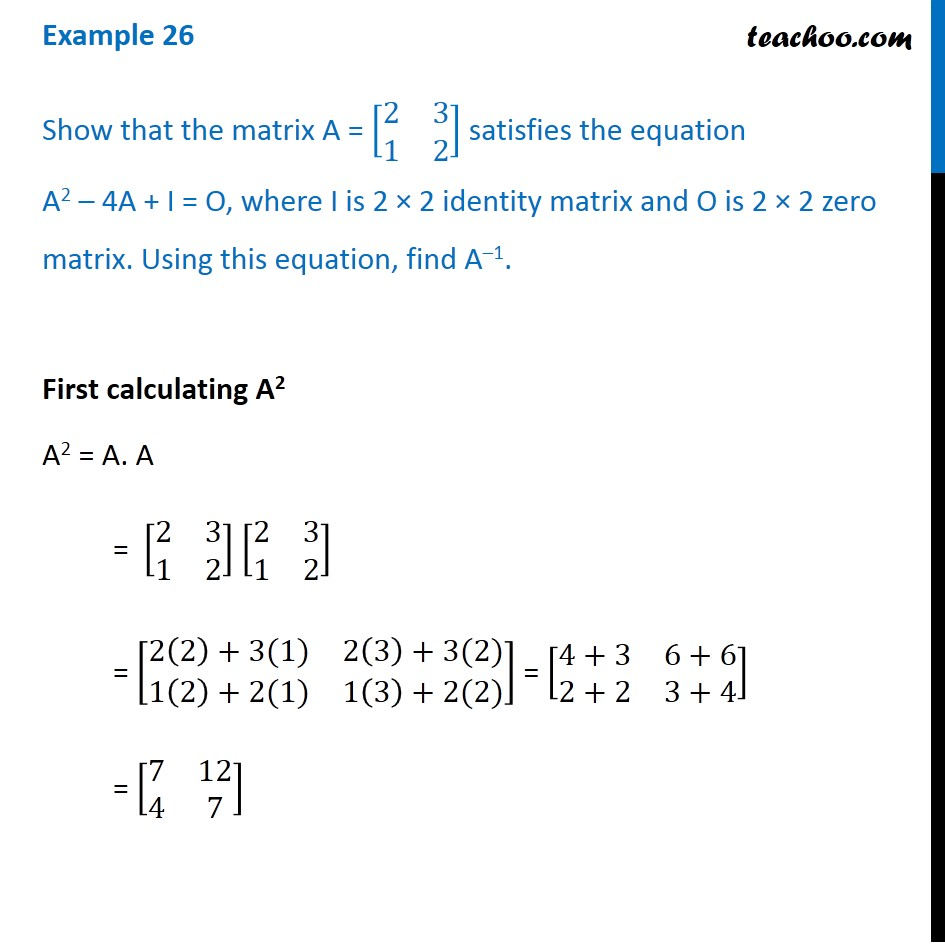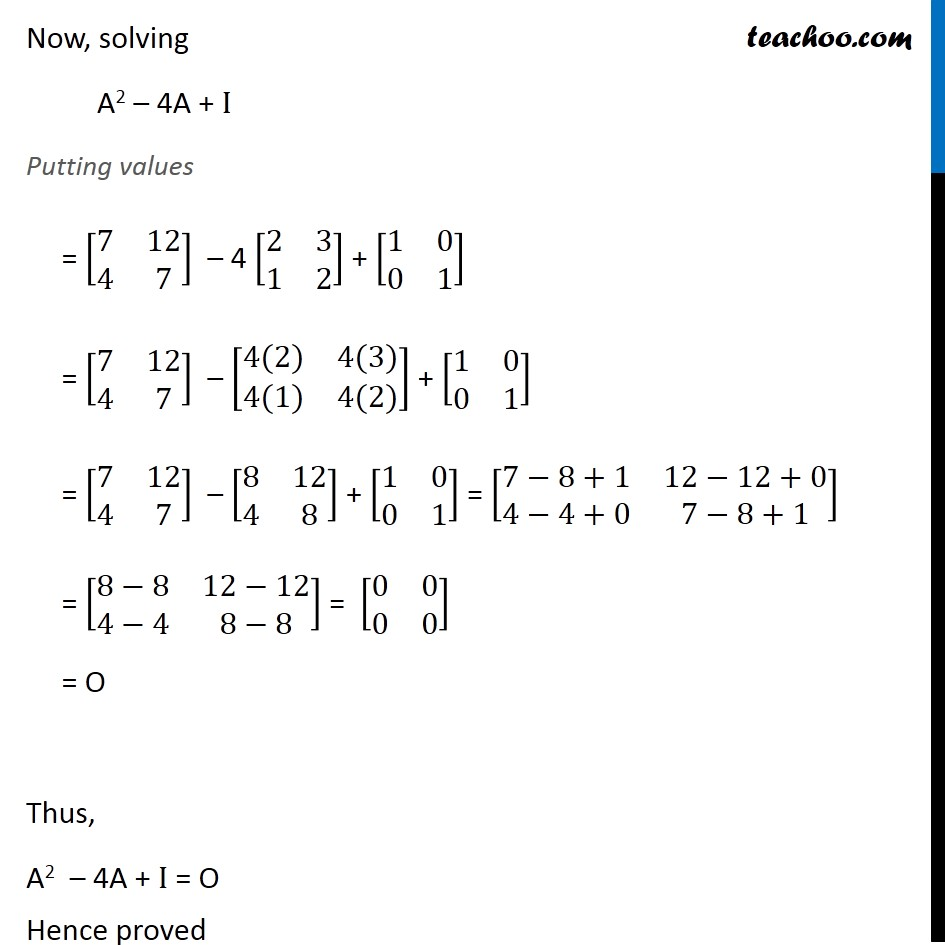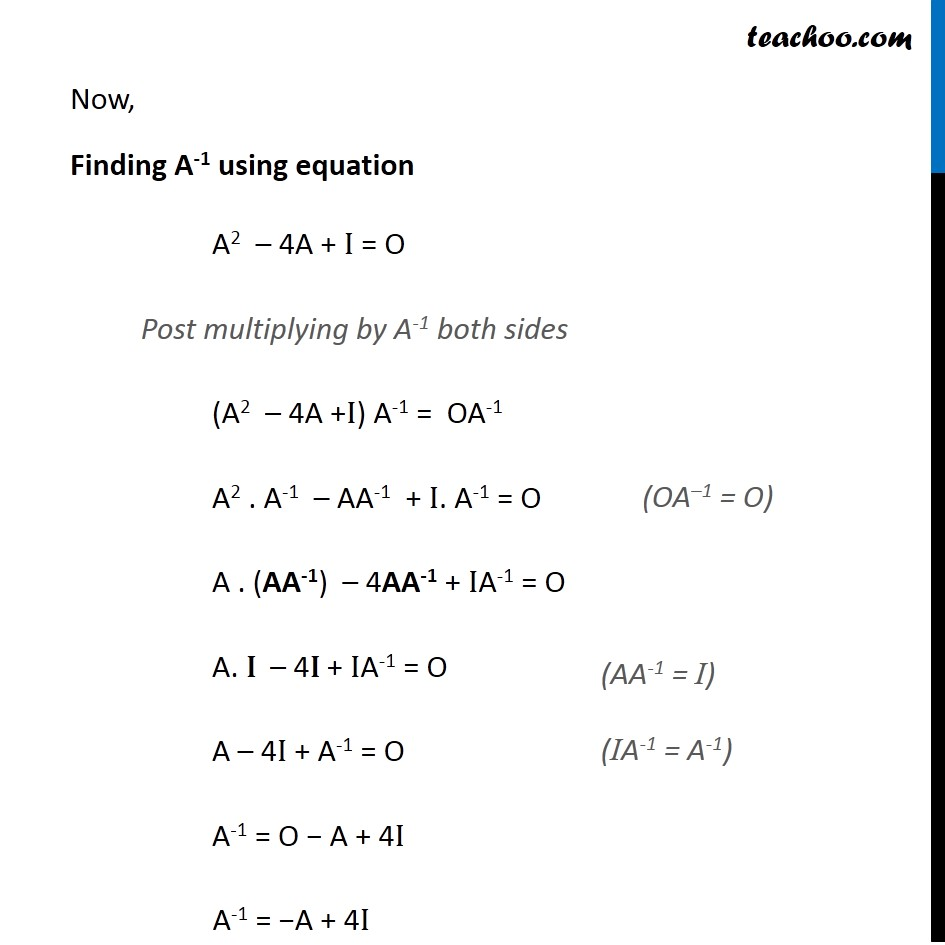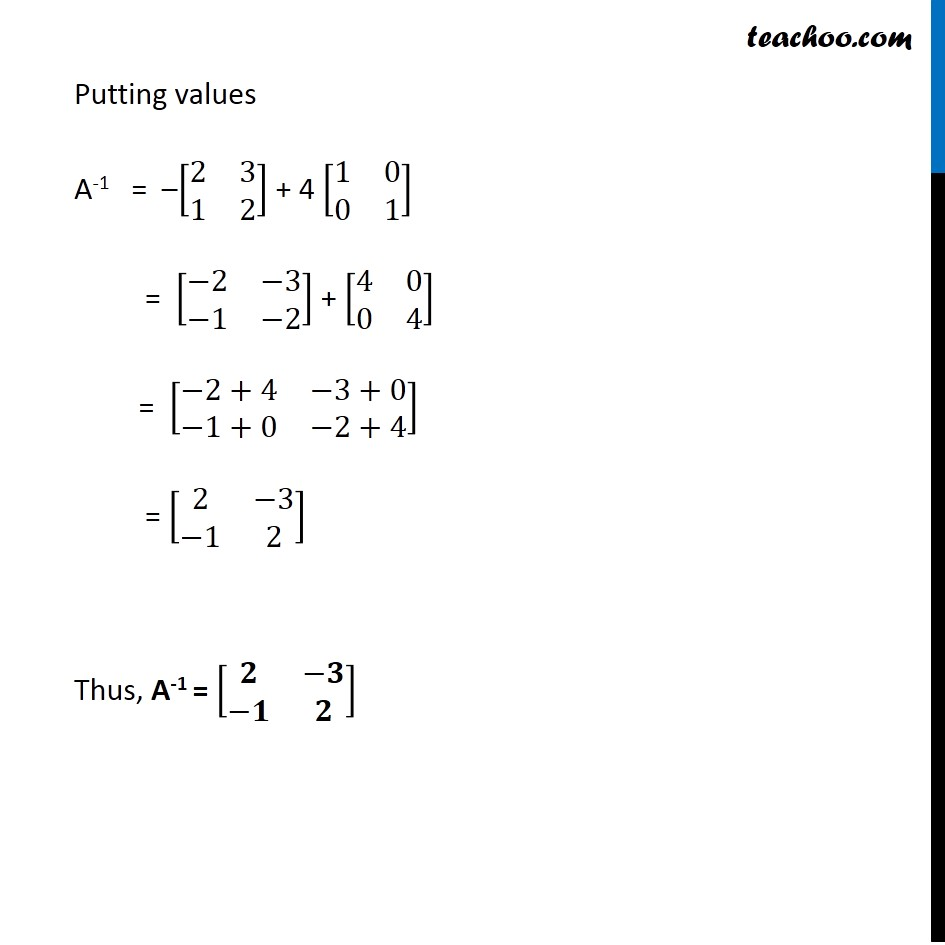Finding inverse when Equation of matrice given

Chapter 4 Class 12 Determinants
Concept wiseIntroducing your new favourite teacher - Teachoo Black, at only ₹83 per month

### Transcript

Example 26 Show that the matrix A = [■8(2&3@1&2)] satisfies the equation A2 – 4A + I = O, where I is 2 × 2 identity matrix and O is 2 × 2 zero matrix. Using this equation, find A–1. First calculating A2 A2 = A. A = [■8(2&3@1&2)] [■8(2&3@1&2)] = [■8(2(2)+3(1)&2(3)+3(2)@1(2)+2(1)&1(3)+2(2))] = [■8(4+3&6+6@2+2&3+4)] = [■8(7&12@4&7)] Now, solving A2 – 4A + I Putting values = [■8(7&12@4&7)] – 4 [■8(2&3@1&2)] + [■8(1&0@0&1)] = [■8(7&12@4&7)] – [■8(4(2)&4(3)@4(1)&4(2))] + [■8(1&0@0&1)] = [■8(7&12@4&7)] – [■8(8&12@4&8)] + [■8(1&0@0&1)] = [■8(7−8+1&12−12+0@4−4+0&7−8+1)] = [■8(8−8&12−12@4−4&8−8)] = [■8(0&0@0&0)] = O Thus, A2 – 4A + I = O Hence proved Now, Finding A-1 using equation A2 – 4A + I = O Post multiplying by A-1 both sides (A2 – 4A +I) A-1 = OA-1 A2 . A-1 – AA-1 + I. A-1 = O A . (AA-1) – 4AA-1 + IA-1 = O A. I – 4I + IA-1 = O A – 4I + A-1 = O A-1 = O − A + 4I A-1 = −A + 4I (OA–1 = O) (AA-1 = I) (IA-1 = A-1) Putting values A-1 = –[■8(2&3@1&2)] + 4 [■8(1&0@0&1)] = [■8(−2&−3@−1&−2)] + [■8(4&0@0&4)] = [■8(−2+4&−3+0@−1+0&−2+4)] = [■8(2&−3@−1&2)] Thus, A-1 = [■8(𝟐&−𝟑@−𝟏&𝟐)]